# Samacheer Kalvi 8th Maths Solutions Term 3 Chapter 1 Numbers Ex 1.2

Students can Download Maths Chapter 1 Numbers Ex 1.2 Questions and Answers, Notes Pdf, Samacheer Kalvi 8th Maths Book Solutions Guide Pdf helps you to revise the complete Tamilnadu State Board New Syllabus and score more marks in your examinations.

## Tamilnadu Samacheer Kalvi 8th Maths Solutions Term 3 Chapter 1 Numbers Ex 1.2

Question 1.
Fill in the blanks:
(i) If a number has 5 or 6 digits in it then, its square root will have………digits.
(ii) The value of 180 lies between integers………and……….
(iii) $$\sqrt{10}$$ × $$\sqrt{6}$$ × $$\sqrt{15}$$ =……………
(iv) $$\frac{\sqrt{300}{\sqrt{192}}$$ =…………….
(v) $$\sqrt{65.61}$$ =…………….
Solution:
(i) 3
(ii) 13, 14
(iii) 30
(iv) $$\frac{5}{4}$$
(v) 8.1Question 2.
Estimate the value of the following square roots to the nearest whole number:
(i) $$\sqrt{440}$$
(ii) $$\sqrt{800}$$
(iii) $$\sqrt{1020}$$
Solution:
(i) We have 20² = 400
21² = 441
∴ $$\sqrt{440}$$  $$\widetilde { – }$$ 21

(ii) We have 28² = 784
29² = 841
∴ $$\sqrt{800}$$ $$\widetilde { – }$$ 28

(iii) We have 31² = 961
32² = 1024
∴ $$\sqrt{1020}$$ $$\widetilde { – }$$ = 32

Question 3.
Find the least number that must be added to 1300 so as to get a perfect square. Also find the square root of the perfect square.
Solution:
We work out the process of finding square root by long division method.
The given number is 1300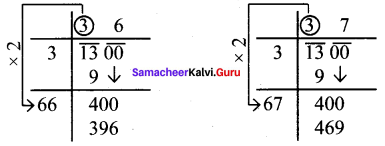So we have 36² < 1300 < 37²
Also 1300 is (469 – 400) = 69 less than 37². So if we add 69 to 1300 it will be perfect square. Hence the required, least number is 69 and the perfect square number is 1300 + 69 = 1369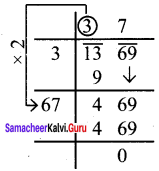∴ $$\sqrt{1369}$$ = 37

Question 4.
Find the least number that must be subtracted to 6412 so as to get a perfect square. Also find the square root of the perfect square.
Solution:
Let us work out the process of finding the square root of 6412 by long division method.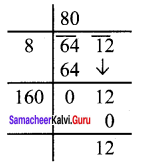The remainder in the last step is 12. Is if 12 be subtracted from the given number the remainder will be zero and the new number will be a perfect square.
∴ The required number is 12.
The square number is 6412 – 12 = 6400
Also $$\sqrt{6400}$$ = 80

Question 5.
Find the square root by long division method.
(i) 17956
(ii) 11025
(iii) 6889
(iv) 1764
(v) 418609
Solution: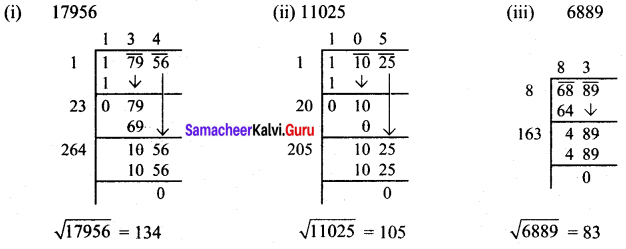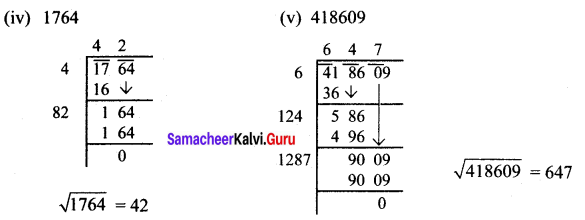Question 6.
Find the square root of the following decimal numbers:
(i) 2.89
(ii) 1.96
(iii) 67.24
(iv) 31.36
(v) 2.0164
(vi) 13.9876
Solution: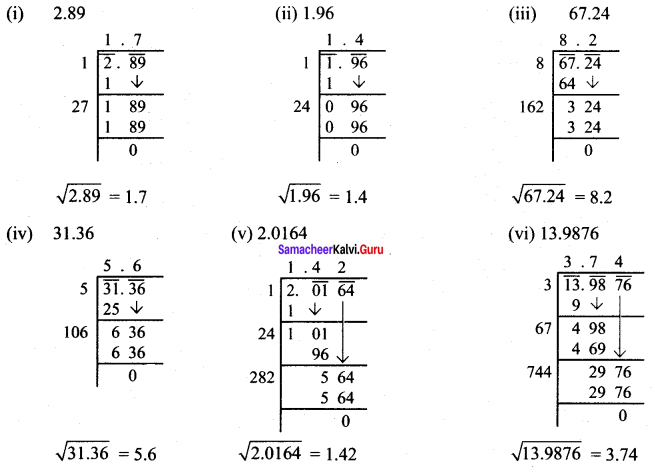Question 7.
Find the square root of each of the following fractions:
(i) $$\frac{144}{225}$$
(ii) 7$$\frac{18}{49}$$
(iii) 6$$\frac{1}{4}$$
(iv) 4$$\frac{25}{36}$$
Solution: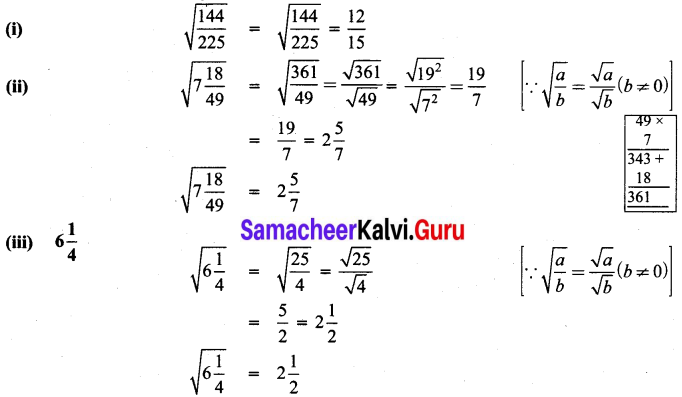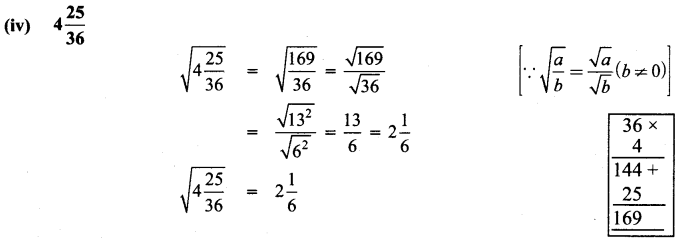Question 8.
Say True or False:
(i) $$\frac{\sqrt{32}}{\sqrt{8}}=2$$
(ii) $$\sqrt{\frac{625}{1024}}=\frac{25}{32}$$
(iii) $$\sqrt{28}{7}=2\sqrt{7}$$
(iv) $$\sqrt{225}{64}=\sqrt{289}$$
(v) $$\sqrt{1 \frac{400}{441}}=1 \frac{20}{21}$$
Solution:
(i) true
(ii) true
(iii) false
(iv) false
(v) false

Objective Type Questions

Question 9.
$$\sqrt{48}$$ is approximately equal to
(a) 5
(b) 6
(c) 7
(d) 8
Solution:
(c) 7
Hint:
$$\sqrt{49}$$ = 7Question 10.
$$\sqrt{128}$$ – $$\sqrt{98}$$ + $$\sqrt{18}$$ =
(a) $$\sqrt{2}$$
(b) $$\sqrt{8}$$
(c) $$\sqrt{48}$$
(d) $$\sqrt{32}$$
Solution:
(d) $$\sqrt{32}$$
Hint:
$$\sqrt{128}-\sqrt{98}+\sqrt{18}=8 \sqrt{2}-7 \sqrt{2}+3 \sqrt{2}=4 \sqrt{2}=\sqrt{32}$$

Question 11.
$$\sqrt{22+\sqrt{7+\sqrt{4}}}=$$
(a) $$\sqrt{25}$$
(b) $$\sqrt{33}$$
(c) $$\sqrt{31}$$
(d) $$\sqrt{29}$$
Solution:
(a) $$\sqrt{25}$$
Hint:
$$\sqrt{22+\sqrt{7+\sqrt{4}}}=\sqrt{22+\sqrt{7+2}}=\sqrt{22+3}=\sqrt{25}$$

Question 12.
The number of digits in the square root of 123454321 is
(a) 4
(b) 5
(c) 6
(d) 7
Solution:
(b) 5
Hint:
$$=\frac{n+1}{2}=\frac{10}{2}=5$$